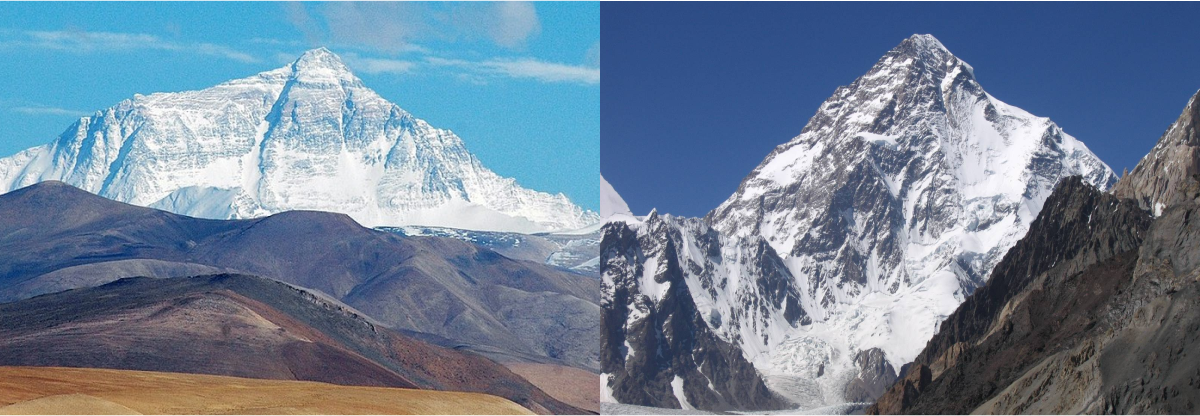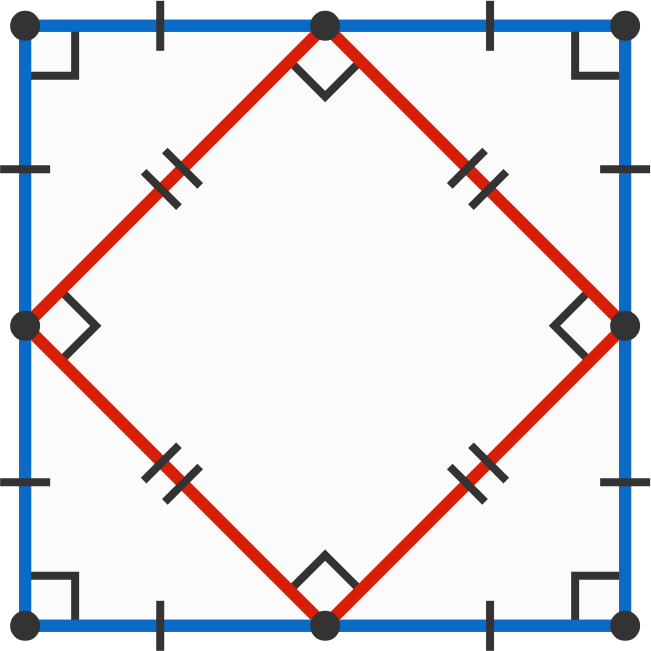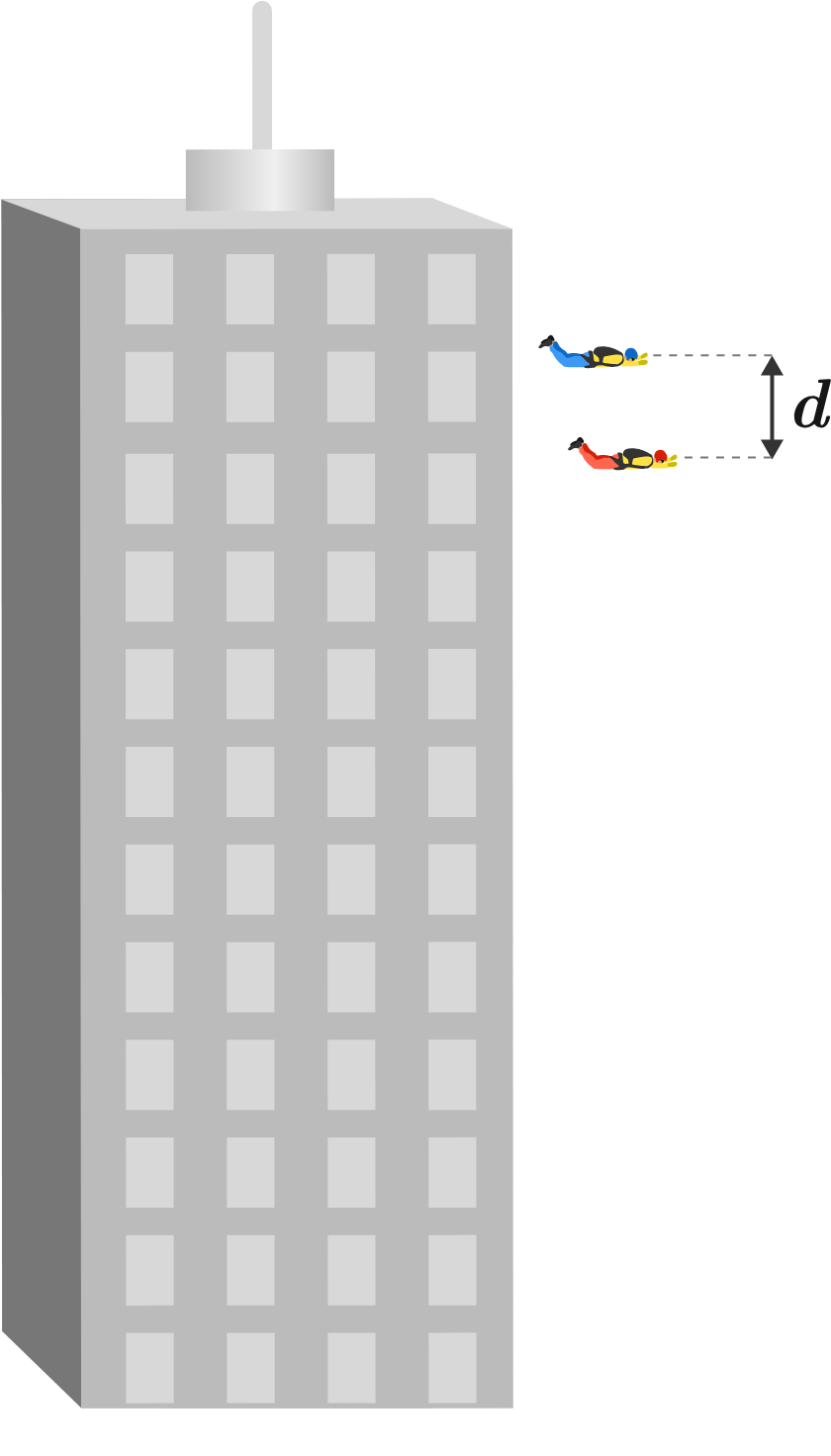# Problems of the Week

Contribute a problem

# 2018-09-24 Basic

Suppose you and a friend set out on separate climbing expeditions, you to Mt. Everest and your friend to Mt. Godwin Austen. You both bring identical cooking equipment and intend to cook rice for dinner with equal volumes of water.

On which peak is the rice going to cook faster?The peak of Mt. Everest (left) is $\SI{8848}{\meter}$ above sea level. The peak of Mt. Godwin Austen (also known as K2, right) is $\SI{8611}{\meter}$ above sea level.A red square is formed when connecting the midpoints of the blue square. What if the blue shape were some other quadrilateral?

Connecting the midpoints of a quadrilateral's four sides forms a square.

Must the original quadrilateral be a square?A villain (in red) jumps from a building, and then $\SI{2}{\second}$ later a superhero (in blue) jumps after him from the same height.

As they're both falling, how does the distance change between them?

Assume that the villain and hero have identical shape and mass.

Randy was exploring the top of a glacier when it suddenly cracked into two pieces, which then started sliding without friction.

How will Randy move, relative to his initial position?Note: This ice is very smooth and slides without friction with other objects (i.e., the ground and Randy).

When $X$ is divided by $Y,$ the remainder is 24.
When $2X$ is divided by $Y,$ the remainder is 11.

What is the value of $Y?$

×